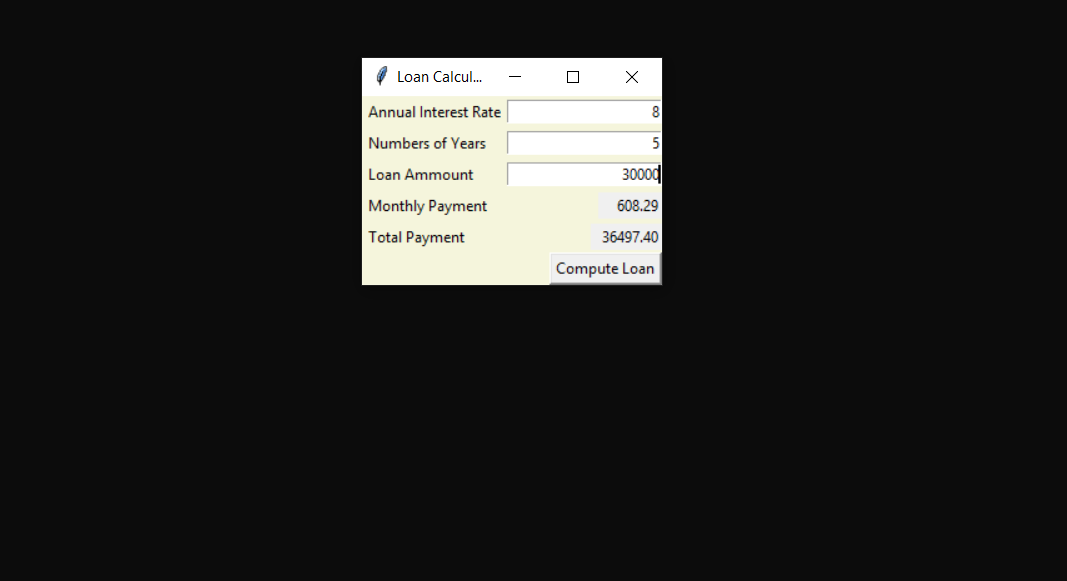## LOAN CALCULATOR IN PYTHON WITH SOURCE CODE

The Loan Calculator In Python is a simple project developed using Python. The project is made for the user convenient, to help them find out about the monthly payments on a specific loan. This project is for financial calculating to figure out the loan’s ...

Type : Project

File Size : 923 bytes# Similar Projects and Reports

#### LOAN CALCULATOR IN PYTHON WITH SOURCE CODE

The Loan Calculator In Python is a simple project developed using Python. The project is made for the user convenient, to help them find out about the monthly payments on a specific loan. This project is for financial calculating to figure out the loan’s ...

#### CALCULATOR IN PYTHON WITH SOURCE CODE

This Calculator project In Python is a simple project developed using Python. The project contains the numbers, operators, and sign like in normal calculator. So, the user can enter any number they want in the calculations. Hence, the user can use a simpl...

#### Calculator App In Python With Source Code

The Calculator App In Python is a simple project developed using Python. The project contains the numbers, operators, and signs like on a normal calculator. So, the user cannot enter the number themselves, they can just click on the numbers they want in t...

#### Python Calculator In GUI With Source Code

The Python Calculator In GUI is a simple project developed using Python. The project contains the numbers, operators, and signs like on a normal calculator. Here, the user can just click on the numbers they want in the calculations. Hence, the user can us...

#### Tip Calculator In Python with Source Code

The Tip Calculator In Python is a simple project developed using Python. The project is for the user convenient, for calculating the tip. This project is an interesting project. The user can calculate the tip amount that they can get from a single bill pa...

#### BMI Calculator In Python With Source Code

The BMI Calculator In Python is a simple project developed using Python. The project is for the user convenient, for checking your BMI. With the BMI value, you can check whether you have a healthy weight or not. The project file contains a python script ...